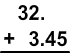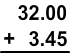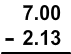# Adding and Subtracting Money Amounts

Print Rate 0 stars Common Core
Lesson size:
Message preview:
Someone you know has shared lesson with you:

To play this lesson, click on the link below:

Hope you have a good experience with this site and recommend to your friends too.

Login to rate activities and track progress.
Login to rate activities and track progress.

When adding and subtracting money, you must always line up the decimal points. If a decimal point is not included in the number, remember that it is understood to be at the end.

Once the decimal points are lined up, you can add or subtract as usual. Just remember to include placeholder zeroes if needed.

Let's take a look at some examples:

## Example 1

We need to line up the decimals. Since there's no decimal written in \$32, it's understood to be at the end.If you want, you can include the placeholder zeroes after \$32.Now add the numbers as usual:Yes, \$32 plus around \$3 should be around \$35, which it is.

## Example 2

What is \$7 minus \$2.13?

We need to line up the decimals.

Remember that since there's no decimal written in \$7, it's understood to be at the end. (That makes sense because \$7 is the same as \$7.00).

Let's also include the placeholder zeroes.Now subtract, borrowing from the 7. Notice that the placeholder zeroes are needed in order to be able to borrow and subtract.

Yes, \$7 minus around \$2 should be around \$5, which our answer (\$4.87) is very close to.

## Example 3

Alex spent \$21.87 on a new toy, \$3.87 on candy, and \$5.18 on a gift for his sister. How much total money did he spend?

We need to line up the decimals:Let's check by estimating: \$22 + \$4 + \$5 = \$31, and our answer is very close to this, so it is reasonable.

## Adding and Subtracting Money Amounts

• When adding and subtracting money, you must always line up the decimal point.
• If a decimal point is not included in the number, remember that it is understood to be at the end.

## Similar Lessons

Become premium member to get unlimited access.